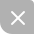# TextRank算法获取文本关键词

### 1.PageRank

• 如果一个网页被很多其他网页链接到的话说明这个网页比较重要，也就是PageRank值会相对较高
• 如果一个PageRank值很高的网页链接到一个其他的网页，那么被链接到的网页的PageRank值会相应地因此而提高

### 2.PageRank算法原理

PageRank算法输出概率分布，用于表示随机点击链接的人到达任何特定页面的可能性。可以为任何大小的文档集合计算PageRank。在一些研究论文中假设在计算过程开始时，分布在集合中的所有文档中均匀分配。PageRank计算需要多次传递，称为“迭代”，通过集合调整近似PageRank值以更接近地反映理论真实值。

P R ( A ) = P R ( B ) + P R ( C ) + P R ( D ) PR(A)=PR(B)+PR(C)+PR(D)

P R ( A ) = P R ( B ) L ( B ) + P R ( C ) L ( C ) + P R ( D ) L ( D ) PR(A)=\frac{PR(B)}{L(B)}+\frac{PR(C)}{L(C)}+\frac{PR(D)}{L(D)}

P R ( u ) = ∑ u ∈ B u P R ( v ) L ( v ) PR(u)=\sum_{u\in B_{u}}^{}\frac{PR(v)}{L(v)}

PageRank通过互联网中的超链接关系来确定一个网页的排名，其公式是通过一种投票的思想来设计的：如果我们要计算网页A的PageRank值（以下简称PR值），那么我们需要知道有哪些网页链接到网页A，也就是要首先得到网页A的入链，然后通过入链给网页A的投票来计算网页A的PR值。这样设计可以保证达到这样一个效果：当某些高质量的网页指向网页A的时候，那么网页A的PR值会因为这些高质量的投票而变大，而网页A被较少网页指向或被一些PR值较低的网页指向的时候,A的PR值也不会很大，这样可以合理地反映一个网页的质量水平。那么根据以上思想，佩奇设计了下面的公式.
S ( V i ) = ( 1 − d ) + d ∗ ∑ u ∈ I n ( V u ) 1 ∣ O u t ( V j ) ∣ S ( V j ) S(V_{i})=(1-d)+d*\sum_{u\in In(V_{u})}^{}\frac{1}{\left | Out(V_{j})\right |}S(V_{j})

### 3.TextRank算法原理

• 如果一个单词出现在很多单词后面的话，那么说明这个单词比较重要。
• 一个TextRank值很高的单词后面跟着的一个单词，那么这个单词的TextRank值会相应地因此而提高。

S ( V i ) = ( 1 − d ) + d ∗ ∑ ( j , i ) ∈ ε w j i ∑ v k ∈ O u t ( V j ) w j k S ( V j ) S(V_{i})=(1-d)+d*\sum_{(j,i)\in \varepsilon }^{}\frac{w_{ji}}{\sum_{v_{k}\in Out(V_{j})}^{}w_{jk}}S(V_{j})

​ TextRank中一个单词i的权重取决于与在i前面的各个点j组成的(j,i)这条边的权重，以及j这个点到其他其他边的权重之和。

### 4.TextRank主要步骤

• 把给定的文本T按照完整句子进行分割；
• 对于每个句子进行分词和词性标注处理，并过滤掉停用词，只保留指定词性的单词，如名词、动词、形容词，即其中是保留后的候选关键词；
• 构建候选关键词图G = (V,E)，其中V为节点集，由（2）生成的候选关键词组成，然后采用共现关系（co-occurrence）构造任两点之间的边，两个节点之间存在边仅当它们对应的词汇在长度为K的窗口中共现，K表示窗口大小，即最多共现K个单词。
• 根据上面公式，迭代传播各节点的权重，直至收敛。
• 对节点权重进行倒序排序，从而得到最重要的T个单词，作为候选关键词。
• 由5得到最重要的T个单词，在原始文本中进行标记，若形成相邻词组，则组合成多词关键词。UP更新不错过~
08-08
01-288125
11-132152
05-183万+
01-07726
01-04
09-19
08-08

### “相关推荐”对你有帮助么？

•非常没帮助
•没帮助
•一般
•有帮助
•非常有帮助被折叠的  条评论 为什么被折叠?到【灌水乐园】发言Atishoo_13

¥2 ¥4 ¥6 ¥10 ¥20余额支付 (余额：-- )扫码支付获取中扫码支付点击重新获取扫码支付1.余额是钱包充值的虚拟货币，按照1:1的比例进行支付金额的抵扣。
2.余额无法直接购买下载，可以购买VIP、C币套餐、付费专栏及课程。余额充值